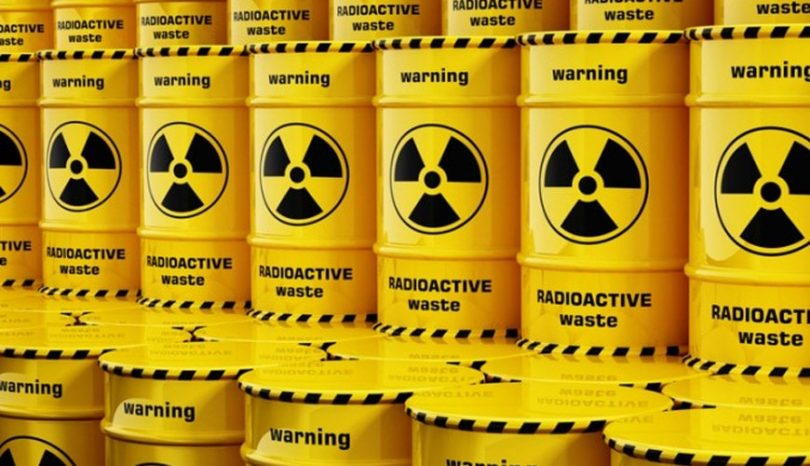# Nuclear Chemistry Quiz### Nuclear Chemistry Practice Questions and Answers:

Ques. Isotones are elements having
(a) Same mass number but different neutrons
(b) Same atomic number but different neutrons
(c) Same atomic number, mass number and neutrons
(d) Different atomic and mass number but same neutrons

Ans. (d)

Ques. Gamma rays are
(a) High energy electromagnetic waves
(b) High energy electrons
(c) High energy protons
(d) Low energy electrons

Ans. (a)

Ques. Nucleons are
(a) Protons and electrons
(b) Protons and neutrons
(c) Electrons and neutrons
(d) Electrons, protons and neutrons

Ans. (b)

Ques. Which particle can be used to change 13Al27 into 15P30
(a) Neutron
(b) alpha-particle
(c) Proton
(d) Deuteron

Ans. (b)

Related: Atomic number practice problems

Ques. If an isotope of hydrogen has two neutrons in its atom, its atomic number and atomic mass number will respectively be
(a) 2 and 1
(b) 3 and 1
(c) 1 and 1
(d) 1 and 3

Ans. (b)

Ques. Which of the following does not characterise X-rays?
(a) The radiation can ionise gases
(b) It causes ZnS to fluorescence
(c) Deflected by electric and magnetic field
(d) Have wavelengths shorter than ultraviolet rays

Ans. (c)

Ques. Formation of nucleus from its nucleons is accompanied by
(a) Decrease in mass
(b) Increase in mass
(c) No change of mass
(d) None of them

Ans. (a)

Ques. 92U235 belongs to group III B of periodic table. If it loses one alpha-particle, the new element will belong to group
(a) I B
(b) I A
(c) III B
(d) V B

Ans. (c)

Related: Electrostatics questions

Ques. Which of the following is radioactive element?
(a) Sulphur
(b) Polonium
(c) Tellurium
(d) Selenium

Ans. (b)

Ques. Radioactive disintegration differs from a chemical change in being
(a) An exothermic change
(b) A spontaneous process
(c) A nuclear process
(d) A unimolecular first order reaction

Ans. (c)

Ques. Meson was discovered
(a) Yukawa
(b) Austin
(c) Moseley
(d) Einstein

Ans. (a)

Ques. Half-life for radioactive C14 is 5760 years. In how many years 200 mg of C14 sample will be reduced to 25 mg
(a) 11520 years
(b) 23040 years
(c) 5760 years
(d) 17280 years

Ans. (d)

Ques. Nuclear theory of the atom was put forward by
(a) Rutherford
(b) Aston
(c) Neils Bohr
(d) J.J. Thomson

Ans. (a)

Ques. Isotopes were discovered by
(a) Aston
(b) Soddy
(c) Thomson
(d) Millikan

Ans. (b)

Ques. The amount of energy, which is required to separate the nucleons from a nucleus. The energy is called
(a) Binding energy
(b) Lattice energy
(c) Kinetic energy
(d) None of these

Ans. (a)

Ques. An isotope of oxygen has mass number 18. Other isotopes of oxygen will have the same
(a) Mass number
(b) Atomic weight
(c) Number of neutrons
(d) Number of protons

Ans. (d)

(a) Stable electronic configuration
(b) Unstable electronic configuration
(c) Stable nucleus
(d) Unstable nucleus

Ans. (d)

Ques. Which of the following properties are different for neutral atoms of isotopes of the same element?
(a) Mass
(b) Atomic number
(c) General chemical reactions
(d) Number of electrons

Ans. (d)

Ques. Which element is the end product of each natural radioactive series?
(a) Sn
(b) Bi
(c) Pb
(d) C

Ans. (c)

Ques. Starting from radium, the radioactive disintegration process terminates when the following is obtained

Ans. (a)

Ques. A freshly prepared radioactive source of half-life 2 hours emits radiations of intensity which is 64 times the permissible safe level. The minimum time after which it would be possible to work safely with this source is
(a) 6 hours
(b) 12 hours
(c) 24 hours
(d) 128 hours

Ans. (b)

Ques. In a radioactive decay, an emitted electron comes from
(a) Nucleus of the atom
(b) Inner orbital of the atom
(c) Outermost orbit of the atom
(d) Orbit having principal quantum number one

Ans. (a)

Ques. If 2.0 g of a radioactive isotope has a half-life of 20 hr, the half-life of 0.5 g of the same substance is
(a) 20 hr
(b) 80 hr
(c) 5 hr
(d) 10 hr

Ans. (a)

Ques. If 3/4 quantity of a radioactive element disintegrates in two hours, its half-life would be
(a) 1 hour
(b) 45 minutes
(c) 30 minutes
(d) 15 minutes

Ans. (a)

Ques. Neutron is used as a
(a) Reducing agent
(b) Moderator
(c) Tracer
(d) In biological programme

Ans. (c)

Ques. In biological programme
(a) Products having a lesser mass than initial substance
(b) Conversion of heavier to lighter atoms
(c) Release of neutrons
(d) Release of electrons

Ans. (a)

Ques. A radioactive isotope has a half-life of 20 days. If 100 gm of the substance is taken, the weight of the isotope remaining after 40 days is
(a) 25 gm
(b) 2.5 gm
(c) 60 gm
(d) 40 gm

Ans. (a)

Ques. Fusion bomb involvesa
(a) Combination of lighter nuclei into bigger nucleus
(b) Destruction of heavy nucleus into smaller nuclei
(c) Combustion of oxygen
(d) Explosion of TNT

Ans. (a)

Ques. Isotopes are atoms having the same
(a) Atomic mass
(b) Mass number
(c) Atomic number
(d) Number of neutrons

Ans. (c)

#### Richa Bhardwaj

Richa (B. Tech) has keen interest in Science and loves to teach students about it through lectures and assignments. She always try to use simple language and sentences while writing to make sure learner understands everything properly.

• Abhijit Rajale says:

Ok